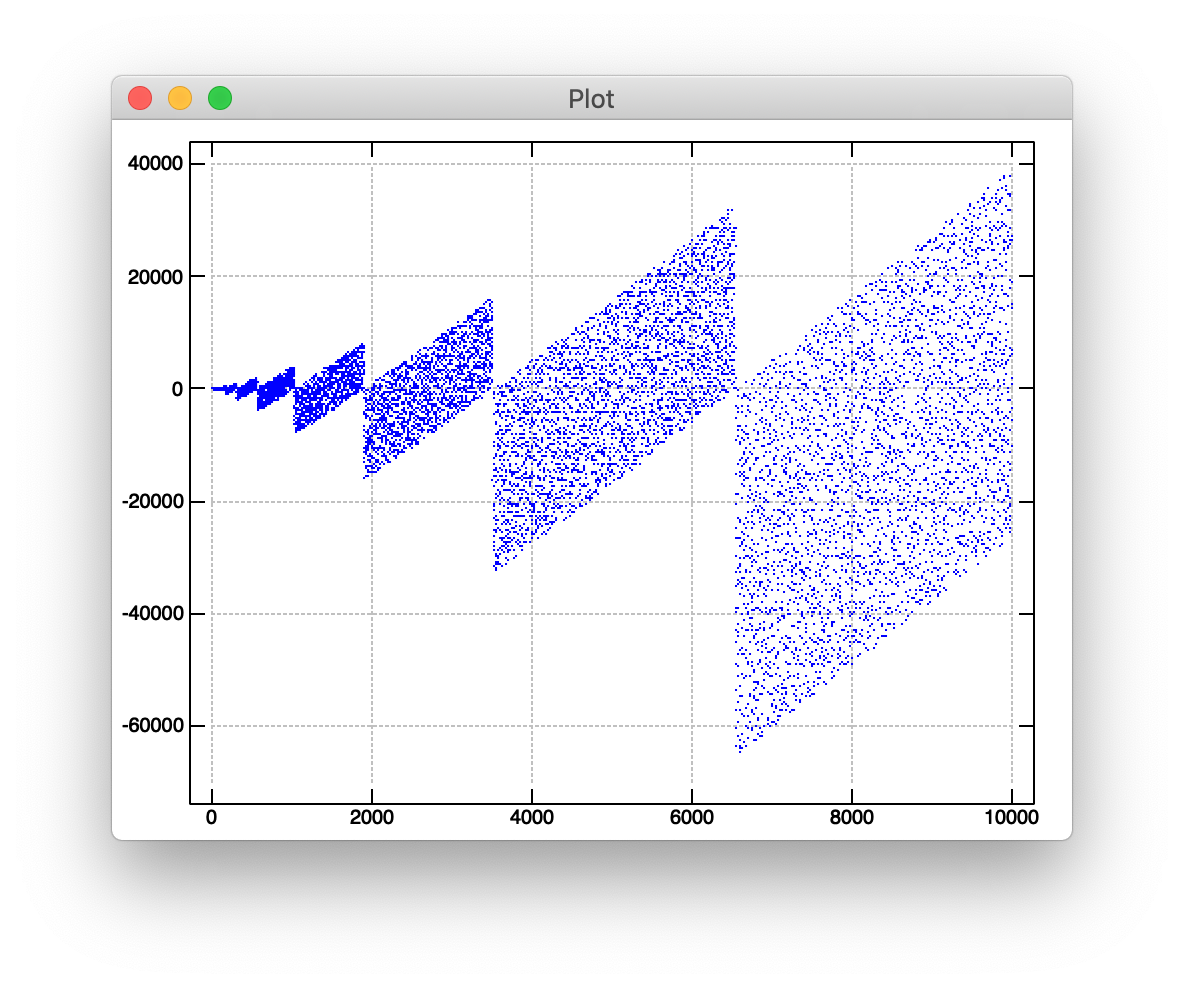A few weeks ago I wrote about an interesting graph of numbers recently investigated by the Numberphile crew. We used it as an opportunity to journey into the world of agendas and gerunds by implementing the graph using the J programming language.

The second half of that same video outlines another interesting number series which has a similarly interesting implementing in J. Let’s try our hand at plotting it.

The basic idea behind calculating the series of numbers in question is to take any positive prime, represent it in base two, reverse the resulting sequence of bits, and subtract the reversed number from the original number in terms of base ten.

Implemented as a tacit, monadic verb in J, this would look something like:

``````
f =: ] - [: #. [: |. #:
``````

Our verb, `f`, is (`=:`) the given number (`]`) minus (`-`) the base two (`#.`) of the reverse (`|.`) of the antibase two (`#:`) of the given number.

We can plot the result of applying `f` to the first ten thousand primes (`p: i. 10000`) like so:

``````
require 'plot'
'type dot' plot f"0 p: i. 10000
``````

If we’re feeling especially terse, we could write this as an almost-one-liner by substituting `f` for our implementation of `f`:

``````
require 'plot'
'type dot' plot (] - [: #. [: |. #:)"0 p: i. 10000
``````Our implementation of `f` is a “train of verbs”, which is to say, a collection of verbs that compose together into hooks and forks. We can visualize this composition by looking at the “boxed” representation of our train:

``````
┌─┬─┬──────────────────┐
│]│-│┌──┬──┬──────────┐│
│ │ ││[:│#.│┌──┬──┬──┐││
│ │ ││  │  ││[:│|.│#:│││
│ │ ││  │  │└──┴──┴──┘││
│ │ │└──┴──┴──────────┘│
└─┴─┴──────────────────┘
``````

From right to left, J greedily groups verbs into three verb forks potentially followed by a final two verb hook if the total number of verbs in the train is even.

We can see that the first fork, `[: |. #:`, is a capped fork, which means it’s roughly equivalent to `|. @: #:`. In the monadic case, this fork takes its argument, converts it to a base two list of ones and zeroes, and reverses that list. Let’s refer to this newly composed verb as `a` moving forward.

The next fork in our train, `[: #. a`, is another capped fork building off of our previous fork. Again, this could be expressed using the `@:` verb to compose `#.` and `a` together: `#. @: a`. In the monadic case, this fork takes its argument, converts it to a reversed binary representation with `a`, and then converts that reversed list of ones and zero back to base ten with `#.`. Let’s call this newly composed verb `b`.

Our final fork, `] - b`, runs our monadic input through `b` to get the base ten representation of our reversed binary, and subtracts it from the original argument.

If we wanted to make J’s implicit verb training explicit, we could define `a`, `b`, and our final `f` ourselves:

``````
a =: [: |. #:
b =: [: #. a
f =: ] - b
``````

But why go through all that trouble? Going the explicit route feels like a natural tendency to me, coming from a background of more traditional programming languages, but J’s implicit composition opens up a world of interesting readability properties.

I’m really fascinated by this kind of composition, and I feel like it’s what makes J really unique. I’ll never pass up an opportunity to try implementing something as a train of verbs.